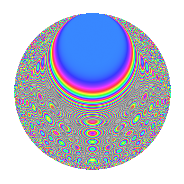# Properties

 Label 630.2.bzLevel 630 Weight 2 Character orbit bz Rep. character $$\chi_{630}(13,\cdot)$$ Character field $$\Q(\zeta_{12})$$ Dimension 192 Newform subspaces 1 Sturm bound 288 Trace bound 0

# Related objects

## Defining parameters

 Level: $$N$$ = $$630 = 2 \cdot 3^{2} \cdot 5 \cdot 7$$ Weight: $$k$$ = $$2$$ Character orbit: $$[\chi]$$ = 630.bz (of order $$12$$ and degree $$4$$) Character conductor: $$\operatorname{cond}(\chi)$$ = $$315$$ Character field: $$\Q(\zeta_{12})$$ Newform subspaces: $$1$$ Sturm bound: $$288$$ Trace bound: $$0$$

## Dimensions

The following table gives the dimensions of various subspaces of $$M_{2}(630, [\chi])$$.

Total New Old
Modular forms 608 192 416
Cusp forms 544 192 352
Eisenstein series 64 0 64

## Trace form

 $$192q + O(q^{10})$$ $$192q + 8q^{11} + 24q^{15} + 96q^{16} - 8q^{18} + 8q^{23} + 48q^{30} - 24q^{35} + 8q^{36} - 44q^{42} + 48q^{46} + 32q^{50} - 160q^{53} - 4q^{56} + 88q^{57} + 24q^{58} + 8q^{60} - 48q^{63} - 8q^{65} + 16q^{71} + 16q^{72} - 16q^{77} - 16q^{78} - 8q^{81} - 16q^{86} + 8q^{92} - 120q^{93} - 128q^{95} - 48q^{98} + O(q^{100})$$

## Decomposition of $$S_{2}^{\mathrm{new}}(630, [\chi])$$ into newform subspaces

Label Dim. $$A$$ Field CM Traces $q$-expansion
$$a_2$$ $$a_3$$ $$a_5$$ $$a_7$$
630.2.bz.a $$192$$ $$5.031$$ None $$0$$ $$0$$ $$0$$ $$0$$

## Decomposition of $$S_{2}^{\mathrm{old}}(630, [\chi])$$ into lower level spaces

$$S_{2}^{\mathrm{old}}(630, [\chi]) \cong$$ $$S_{2}^{\mathrm{new}}(315, [\chi])$$$$^{\oplus 2}$$

## Hecke Characteristic Polynomials

There are no characteristic polynomials of Hecke operators in the database